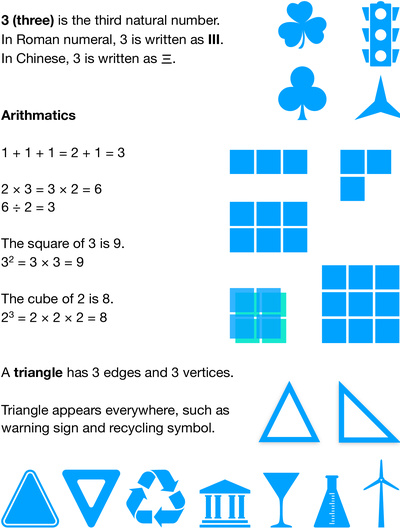# All about the number 3Early math skills are developed through daily routines and activities. The basics of math are numbers and simple arithmetics. By age 5 to 7 years, children are beginning able to make simple arithmetic calculations. This worksheet focuses on the number 3, and shows some simple arithmetics involving 3, including addition, multiplication division, square, and cube. This worksheet also presents triangle, that is, the basic shape with three edges and three vertices. It also helps kids recognizing triangles in real world objects such as road signs, building, and various daily used tools.